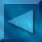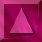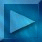Previous: The modified Geman-Yang...  Next : The reconstruction algorithm Notations

 The MCMCML estimation algorithm

"Markov Chain Monte Carlo Maximum Likelihood"

Minimization of the -log likelihood :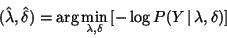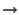Descent method : we use the gradient of the criterion w.r.t.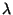and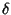.is fixed and we only compute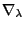, the gradient of the criterion w.r.t..is fixed because for eachwe can find a value offor which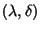is optimal.Estimation and restoration processes are simultaneous :
(the modified Geman-Yang sampler is initialized with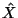)

 The MCMCML estimation algorithm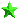Initialization :  -chosen in order to penalize the gradients due to noise     and to preserve edges.   - The ratio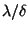corresponds to the best Wiener filter.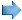Compute:is reconstructed from Y with the ICM-DCT algorithm,   with the current couple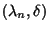.Compute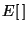and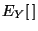:  Generate 2 Markov chains with the Modified Geman-Yang algorithm :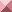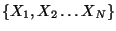sampled from the prior modeletc.sampled from the posterior distributionetc.  Compute the empirical mean :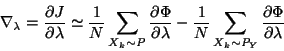Iteration from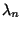to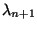: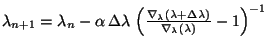where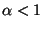(to  ensure convergence)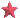Stop criterion :  We stop the algorithm if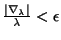where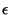is the estimation error (%) fixed at the beginning.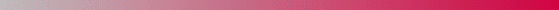André Jalobeanu - 24 Aug 1998